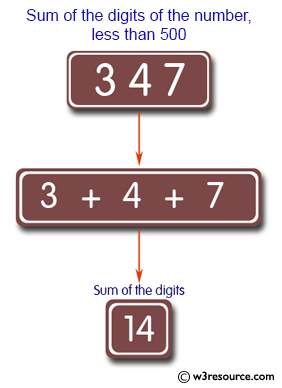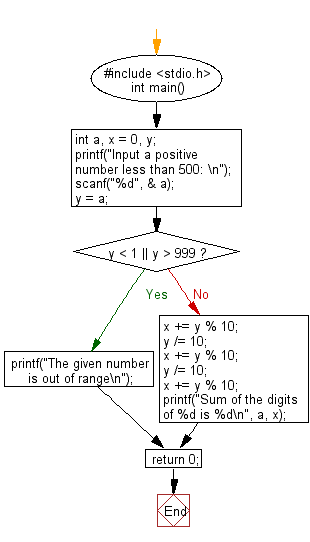﻿ C : Sums the digits of a positive integer less than 500

# C Exercises: Accepts a positive integer less than 500 and prints out the sum of the digits of this number

## C Basic Declarations and Expressions: Exercise-62 with Solution

Write a C program that accepts a positive integer less than 500 and prints out the sum of the digits of this number.

Test data and expected output:
Input a positive number less than 500:
Sum of the digits of 336 is 12

Pictorial Presentation:Sample Solution:

C Code:

``````#include <stdio.h>
int main() {
int a, x = 0, y;

// Prompt user for input
printf("Input a positive number less than 500: \n");

scanf("%d", &a);

y = a;

if (y < 1 || y > 999) {
// Display error message if the number is out of range
printf("The given number is out of range\n");
} else {
// Calculate the sum of the digits
x += y % 10;
y /= 10;
x += y % 10;
y /= 10;
x += y % 10;

// Display the result
printf("Sum of the digits of %d is %d\n", a, x);
}

return 0;
}
``````

Sample Output:

```Input a positive number less than 500:
Sum of the digits of 347 is 14
```

Flowchart:C programming Code Editor:

What is the difficulty level of this exercise?

Test your Programming skills with w3resource's quiz.

﻿